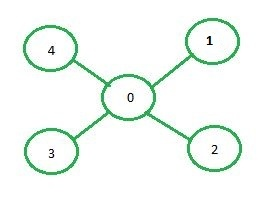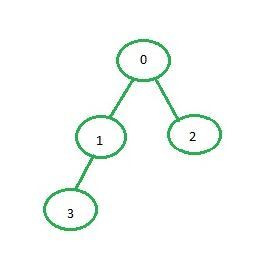Related Articles
Implementation of DFS using adjacency matrix
• Difficulty Level : Easy
• Last Updated : 19 Aug, 2020

Adjacency matrix representation: In adjacency matrix representation of a graph, the matrix mat[][] of size n*n (where n is the number of vertices) will represent the edges of the graph where mat[i][j] = 1 represents that there is an edge between the vertices i and j while mat[i][i] = 0 represents that there is no edge between the vertices i and j.Below is the adjacency matrix representation of the graph shown in the above image:

```   0 1 2 3 4
0  0 1 1 1 1
1  1 0 0 0 0
2  1 0 0 0 0
3  1 0 0 0 0
4  1 0 0 0 0
```

Examples:

```Input: source = 0Output: 0 1 3 2

Input: source = 0Output: 0 1 2 3 4
```

## Recommended: Please try your approach on {IDE} first, before moving on to the solution.

Approach:

• Create a matrix of size n*n where every element is 0 representing there is no edge in the graph.
• Now, for every edge of the graph between the vertices i and j set mat[i][j] = 1.
• After the adjacency matrix has been created and filled, call the recursive function for the source i.e. vertex 0 that will recursively call the same function for all the vertices adjacent to it.
• Also, keep an array to keep track of the visited vertices i.e. visited[i] = true represents that vertex i has been been visited before and the DFS function for some already visited node need not be called.

Below is the implementation of the above approach:

## C++

 `// C++ implementation of the approach ` `#include ` `using` `namespace` `std; ` ` `  `class` `Graph { ` ` `  `    ``// Number of vertex ` `    ``int` `v; ` ` `  `    ``// Number of edges ` `    ``int` `e; ` ` `  `    ``// Adjacency matrix ` `    ``int``** adj; ` ` `  `public``: ` `    ``// To create the initial adjacency matrix ` `    ``Graph(``int` `v, ``int` `e); ` ` `  `    ``// Function to insert a new edge ` `    ``void` `addEdge(``int` `start, ``int` `e); ` ` `  `    ``// Function to display the DFS traversal ` `    ``void` `DFS(``int` `start, vector<``bool``>& visited); ` `}; ` ` `  `// Function to fill the empty adjacency matrix ` `Graph::Graph(``int` `v, ``int` `e) ` `{ ` `    ``this``->v = v; ` `    ``this``->e = e; ` `    ``adj = ``new` `int``*[v]; ` `    ``for` `(``int` `row = 0; row < v; row++) { ` `        ``adj[row] = ``new` `int``[v]; ` `        ``for` `(``int` `column = 0; column < v; column++) { ` `            ``adj[row][column] = 0; ` `        ``} ` `    ``} ` `} ` ` `  `// Function to add an edge to the graph ` `void` `Graph::addEdge(``int` `start, ``int` `e) ` `{ ` ` `  `    ``// Considering a bidirectional edge ` `    ``adj[start][e] = 1; ` `    ``adj[e][start] = 1; ` `} ` ` `  `// Function to perform DFS on the graph ` `void` `Graph::DFS(``int` `start, vector<``bool``>& visited) ` `{ ` ` `  `    ``// Print the current node ` `    ``cout << start << ``" "``; ` ` `  `    ``// Set current node as visited ` `    ``visited[start] = ``true``; ` ` `  `    ``// For every node of the graph ` `    ``for` `(``int` `i = 0; i < v; i++) { ` ` `  `        ``// If some node is adjacent to the current node ` `        ``// and it has not already been visited ` `        ``if` `(adj[start][i] == 1 && (!visited[i])) { ` `            ``DFS(i, visited); ` `        ``} ` `    ``} ` `} ` ` `  `// Driver code ` `int` `main() ` `{ ` `    ``int` `v = 5, e = 4; ` ` `  `    ``// Create the graph ` `    ``Graph G(v, e); ` `    ``G.addEdge(0, 1); ` `    ``G.addEdge(0, 2); ` `    ``G.addEdge(0, 3); ` `    ``G.addEdge(0, 4); ` ` `  `    ``// Visited vector to so that ` `    ``// a vertex is not visited more than once ` `    ``// Initializing the vector to false as no ` `    ``// vertex is visited at the beginning ` `    ``vector<``bool``> visited(v, ``false``); ` ` `  `    ``// Perform DFS ` `    ``G.DFS(0, visited); ` `} `

## Python3

 `# Python3 implementation of the approach  ` `class` `Graph: ` `     `  `    ``adj ``=` `[] ` ` `  `    ``# Function to fill empty adjacency matrix ` `    ``def` `__init__(``self``, v, e): ` `         `  `        ``self``.v ``=` `v ` `        ``self``.e ``=` `e ` `        ``Graph.adj ``=` `[[``0` `for` `i ``in` `range``(v)]  ` `                        ``for` `j ``in` `range``(v)] ` ` `  `    ``# Function to add an edge to the graph ` `    ``def` `addEdge(``self``, start, e): ` `         `  `        ``# Considering a bidirectional edge ` `        ``Graph.adj[start][e] ``=` `1` `        ``Graph.adj[e][start] ``=` `1` ` `  `    ``# Function to perform DFS on the graph ` `    ``def` `DFS(``self``, start, visited): ` `         `  `        ``# Print current node ` `        ``print``(start, end ``=` `' '``) ` ` `  `        ``# Set current node as visited ` `        ``visited[start] ``=` `True` ` `  `        ``# For every node of the graph ` `        ``for` `i ``in` `range``(``self``.v): ` `             `  `            ``# If some node is adjacent to the  ` `            ``# current node and it has not  ` `            ``# already been visited ` `            ``if` `(Graph.adj[start][i] ``=``=` `1` `and` `                    ``(``not` `visited[i])): ` `                ``self``.DFS(i, visited) ` ` `  `# Driver code ` `v, e ``=` `5``, ``4` ` `  `# Create the graph ` `G ``=` `Graph(v, e) ` `G.addEdge(``0``, ``1``) ` `G.addEdge(``0``, ``2``) ` `G.addEdge(``0``, ``3``) ` `G.addEdge(``0``, ``4``) ` ` `  `# Visited vector to so that a vertex ` `# is not visited more than once ` `# Initializing the vector to false as no ` `# vertex is visited at the beginning ` `visited ``=` `[``False``] ``*` `v ` ` `  `# Perform DFS ` `G.DFS(``0``, visited); ` ` `  `# This code is contributed by ng24_7 `

Output:

```0 1 2 3 4
```

Attention reader! Don’t stop learning now. Get hold of all the important DSA concepts with the DSA Self Paced Course at a student-friendly price and become industry ready.

My Personal Notes arrow_drop_up
Recommended Articles
Page :